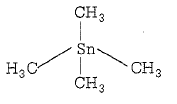NEET and AIPMT NEET Chemistry Coordination Compounds MCQ Questions Solved

In which of the following coordination entities the magnitude of $∆$0(CFSE in octahedral field)will be maximum?

(a) [Co(H2O)6]3+

(b) [Co(NH3)6]3+

(c) [Co(CN)6]3-

(d) [Co(C2O4)3]3-

(c) As in all the given complex the central metal atom is same and contains same number of d electrons, thus CFSE is decided by ligands. In case of strong field ligand, CFSE is maximum. CN- is a strong filed ligand,Hencem in [Co(CN)6]3- CFSE is maximum.The magnitude of $∆$oct will be maxmimum in [Co(CN)6]3-

Difficulty Level:

• 16%
• 23%
• 51%
• 12%

Fe2(CO)9 is diamagnetic.Which of the following reasons is correct?

(a) Presence of one CO as bridge group

(b) Presence of monodentate ligand

(c) Metal-metal(Fe-Fe) bond in molecule

(d) Resonance hybridization of CO

(C) Metal-metal(Fe-Fe) bond pair up the unpaired electrons

Difficulty Level:

• 25%
• 24%
• 33%
• 20%

From the stability constant (hypothetical  values)given below, predict which is the strongest ligand?

(a) Cu2++4NH3$⇌$[Cu(NH3)4]2+;(k=4.5x1011)

(b) Cu2++4CN$⇌$[Cu(CN)4]2-;(K=2.0x1027)

(c) Cu2++2en$⇌$[Cu(en)2]2+;(K=3.0x1015)

(d) Cu2++4H2O$⇌$[Cu(H2O)4]2+;(K=9.5x108)

(b) Higher is the stability constant of ligand, lesser is its dissociation,more is its stability

Difficulty Level:

• 8%
• 67%
• 13%
• 14%

Which does not obey EAN rule?

(a) [Cu(NH3)4]2+

(b) [Zn(OH)4]2-

(c) [HgI4]2-

(d) Fe(CO)5

(a) The EAN for Cu in [Cu(NH3)4]2+ is 35 and not 36, the next inert gas at. no.

Difficulty Level:

• 25%
• 18%
• 31%
• 28%

The coordination number and oxidation state of Cr in K3[Cr(C2O4)3] are respectively

(a) 3 and +3

(b) 3 and 0

(c) 6 and +3

(d) 4 and +2

(c) Coordination number of Cr is 6(oxalte is bidentate ligand)and oxidation state of Cr in

K3[Cr(C2O4)3]

3(1)+x+3(-2)=0

3+x+(-6)=0

x=6-3

x=+3

Difficulty Level:

• 26%
• 16%
• 57%
• 3%

Which one of the following octahedral complexes will not show geometrical isomerism?(A and B are monodentate ligands)

(a) [MA4B2]

(b) [MA5B]

(c) [MA2B4]

(d) [MA3B3]

(b) [MA5B] due to absence of symmetry of B cannot exist in the form of cis-trans-isomer.

Difficulty Level:

• 9%
• 71%
• 10%
• 13%

According to IUPAC nomenclature sodium nitroprusside is named as

(a) sodium pentacyanonitrosyl ferrate(II)

(b) sodium pentacyanonitrosyl ferrate(III)

(c) sodium nitroferricyanide

(d) sodium nitroferrocyanide

(b) IUPAC name of sodium nitroprusside Na2[Fe(CN)5NO] is sodium pentacyanoni trosyl ferrate(III) beacuse in it NO is neutral ligand and the oxidation number of Fe is +3. Which is calculated as

Na2[Fe(CN)5NO]

2x(+1)+X+5x(-1)+1x0=0

2+X-5=0

X-3=0

X=+3

Difficulty Level:

• 27%
• 48%
• 18%
• 9%

Amoung the following which is not the $\mathrm{\pi }$-bonded organomettalic compound?

(a) K[PtCl3(${\eta }^{\mathit{2}}$-C2H4)]

(b) Fe(${\eta }^{5}$-C2H5)2

(c) Cr(${\eta }^{6}$-C6H6)2

(d) (CH3)4Sn

(d) In (CH3)4 Sn(organometallic compounds of tin) single bonds are present in form of sigma bond. pi-binded organometallic compound includes organometallic compound includes organometallic compounds of alkenes,alkynes and some other carbon containing compounds having pi-electrons in their molecular orbitals.Difficulty Level:

• 13%
• 18%
• 10%
• 61%

IUPAC name of [Pt(NH3)3 (Br)(NO2)Cl]Cl is

(a) triamminebromochloronitroplatinum(IV)Chloride

(b) triamminebromonitrocholoroplatinum(IV)Chloride

(c) triamminechlorobromonitroplatinum(IV)Chloride

(d)triamminenitrochlorobromoplatinum(IV)Chloride

(a) The IUPAC name of [Pt(NH3)3 (Br)(NO2)Cl]Cl is triamminebromochloronitroplatinum(IV)Chloride

(Oxidation number of Pt=+4, and ligands are arranged in alphabetical order)

Difficulty Level:

• 70%
• 18%
• 9%
• 4%

The equation which is balanced and represents the correct product(s) is:

(a) Li2O +2KCl$\to$2LiCl+K2O

(b) [CoCl(NH3)5]++5H+$\to$Co2++5NH4++Cl-

(c) [Mg(H2O)6]2+ +(EDTA)4-[Mg(EDTA)]2++6H2O

(d) CuSO4+4KCN$\to$K2[Cu(CN)4+K2SO4

(b) In a,c,d changes are wrongly reported:

(a) 2LiCl+K2O$\to$Li2O+2KCl

(c) [Mg(H2O)6]2++EDTA4-$\stackrel{\mathrm{OH}-}{\to }$[Mg(EDTA)2-+6H2O

(d) [CoCl(NH3)5]++5H+$\to$Co2++5NH4++Cl-

Difficulty Level:

• 21%
• 22%
• 26%
• 33%